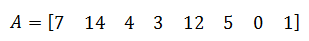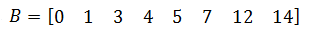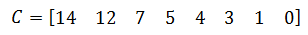Home MathLab Sort a Matrix in Matlab

# Sort a Matrix in Matlab

Sorting Matrices in Matlab is one of the easiest things to do when you happen to know the language to use to order the software to do so.

In this example, we will deal with vectors, and we will write simple programs sorting a vector in the ascending and descending order all for the objective to make you understand the basics about sorting in Matlab.

## Sort a Matrix in Matlab

There exist an inbuilt Matlab function that does sort Matrices for you, all you have to do is call it when needed.

Let’s consider the following vectorSorting A in ascending order will result toand sorting A in descending order will result toLet’s see how that works in Matlab

Matlab code

[php]A=[7 14 4 3 12 5 0 1];
B=sort(A) % You can also use B=sort(A,’ascend’)
C=sort(A,’descend’)[/php]

As simple as that.

let’s work more with a couple of examples, maybe those help practice you Matlab skills

## Example 1

Let’s write a small program that sorts any matrix of 5 elements. We want to give the possibility to anyone to enter 5 elements, we will then put those elements in a matrix, sort them and display the result.

Step 1: Ask element after element 5 times, and each time, but the element in the matrix A, from position 1 to position 5

Step 2: sort the Matrix

Step 3: display the sorted matrix

Matlab code

[php]for i=1:5
A(i)=input(‘Enter a number ‘);
end
B=sort(A);
B[/php]

## Example 2

In the previous example, we predefined the number of elements of the vector, not giving an option to users to use a vector with either more or less number of elements than 5. Now we want to sort any vector. What we do is, ask the user to provide the length of his vector first, so as to be able to manipulate the for loop.

Matlab code

[php]length=input(‘What is the length of your vector ‘);
for i=1:length
A(i)=input(‘Enter a number ‘);
end
B=sort(A);
B[/php]

As basic as this can be, we hope it has given you a glimpse of how you can play with for loops in Matlab, and more importantly how you can sort matrices.